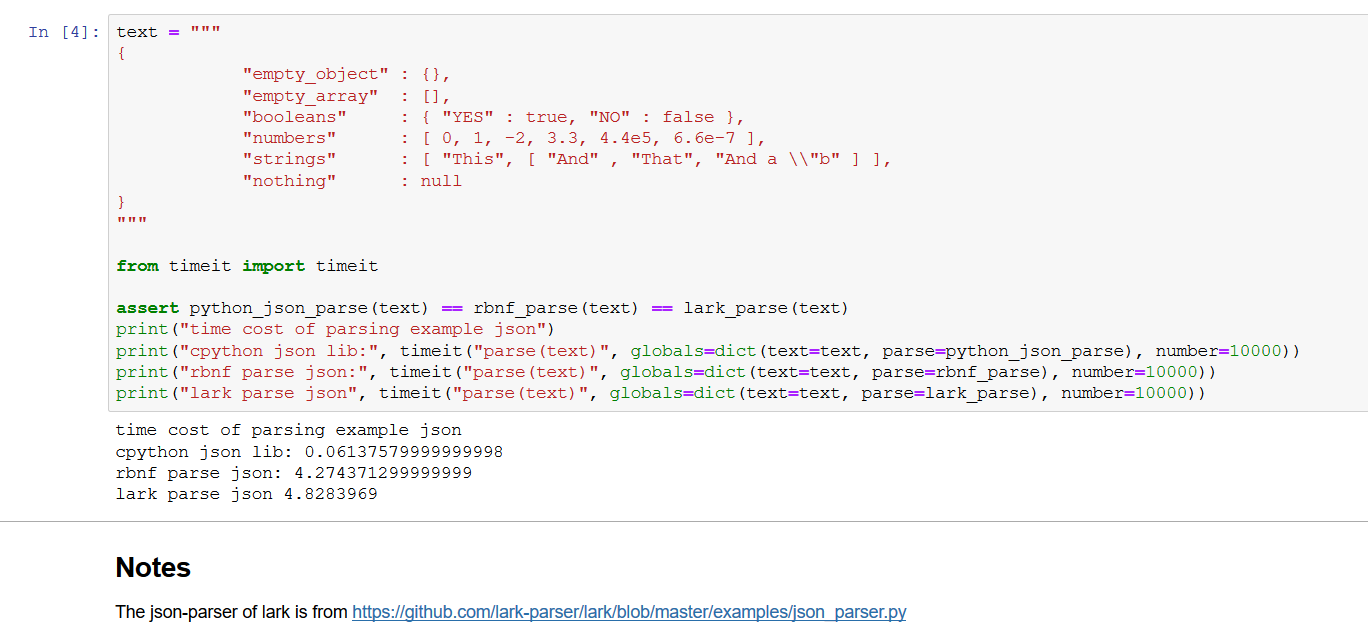No project description provided

# rbnf-rts

Runtime support for generated parsers of RBNF.hs

P.S:

rbnf-rts is the new fastest Python parser generator by 2020, according to a benchmark given by benchmark-json.## Features

• Syntax-driven
• Left recursion with LL parsing(yes, left recur in LL, it's right)
• Grammar inline optimizations
• Smarter lookahead via ID3 algorithm
• Statically generated(no need to create the parser again and again when starting your application)
• Good error reports(including the position and nested rule names of parsing error)
• RBNF.hs is language independent
• [WIP]: context sensitive parsing as an extension to CFG

## More Examples

Check the test directory:

• test
• multiply : parser/lexer implementation for multiplications
• arith : parser/lexer implementation for arithmetics
• relax : parser/lexer implementation for a full-featured programming language
• llvmir: parser/lexer implementation for LLVM IR, nearly full-featured
• rbnfjson: a json parser implemented by RBNF.hs and rbnf-rts

In each sub-directory of test, you can run tests via directly invoking the test.sh., like cd test/llvmir && bash test.sh

## Native Dependencies

• The executable rbnf-pgen in PATH.

If ~/.local/bin is already in PATH:

git clone https://github.com/thautwarm/RBNF.hs
cd RBNF.hs
stack install .


## Example: Multiplications

1. write a multiply.exrbnf file
# 'mul' is a python global which should be marked as 'required' in .rlex
Mul    : lhs=Mul "*" rhs=Atom { mul(lhs, rhs) }
| a=Atom  {a}
;

# 'unwrap' should be marked as 'required', just as 'mul'
Atom   :  "(" !a=Mul ")"  {a} ;
|  <number>        { unwrap($0) }; START ::= <BOF> Mul <EOF> {$1 };


or write a multiply.rbnf file:

# 'mul' is a python global which should be marked as 'required' in .rlex
Mul    : !lhs=Mul "*" !rhs=Atom            -> mul(lhs, rhs);
Mul    : !a=Atom                           -> a;
Atom   : "(" !a=Mul ")"                    -> a;

# 'unwrap' should be marked as 'required', just as 'mul'
Atom   : !a=<number>                       -> unwrap(a);
START ::= <BOF> !a=Mul <EOF>               -> a;

1. write a multiply.rlex file:
%require mul
%require unwrap
%ignore space
number [+-]?\d+
space \s+

1. codegen
sh> rbnf-pygen multiply.rbnf multiply.rlex multiply.py --k 1 --traceback

1. run statically-generated parsers and lexers and enjoy its efficiency
from rbnf_rts.rts import Tokens, State
from multiply import run_lexer, mk_parser
import operator

def unwrap(x: Token):
return int(x.value)

scope = dict(mul=operator.mul, unwrap=unwrap)

parse = mk_parser(**scope)

tokens = list(run_lexer("<current file>", "-1 * 2 * (3 * 4)"))
got = parse(State(), Tokens(tokens))
print(got)


Got (True, -24), where True indicates the parsing succeeded.

If False, a list of errors will be given in the second element of the return tuple.

1. Menhir-like syntax sugars including list and separated_list.

A json parser more than 20% efficient than that of lark-parser(lol), written as a .exrbnf file.

START: <BOF> value <EOF> { $1 }; value: <ESCAPED_STRING> { DQString(*$0) }
| <SIGNED_INT>     { int(*$0) } | <SIGNED_FLOAT> { float(*$0) }
| "true" { True }
| "null" { None }
| "false" { False }
# array
| '[' ']' {[]}
| '[' separated_list(',', value) ']' { $1 } # object | '{' '}' { dict() } | '{' separated_list(',', pair) '}' { dict($1) }
;
pair   : <ESCAPED_STRING> ":" value { (DQString(*$0),$2) };


## Project details

This version1.00.420.410.70.6.10.60.5.10.50.40.30.20.1

Uploaded py3